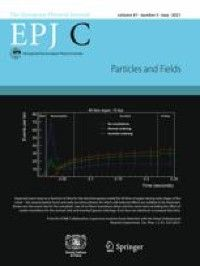A tachyon field might be responsible for cosmological inflation at an early time and contribute to cosmological dark matter at a later time. We investigate tachyonic inflation by analyzing a tachyon field with different potentials in the framework of loop quantum cosmology. No matter which tachyon field energy dominates at the bounce, the evolution of the background can be divided into three phases: super-inflation, damping, and slow-roll inflation. The duration of each phase depends on the initial condition. During the slow-roll inflation, when the initial condition is $$V(T_\mathrm{B})/\rho _\mathrm{c}\ge 10^{-6}$$ V(TB)/ρc≥10–6, the number of e-folds is very high ($$N\gg 60$$ N≫60) for $$V\propto T^{-n}$$ V∝T-n with $$n=1$$ n=1 and 1 / 2. For an exponential potential, to get enough e-folds, $$V(T_\mathrm{B})/\rho _\mathrm{c}$$ V(TB)/ρc should be greater than $$7.802\times 10^{-4}$$7.